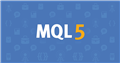# How to get time in 24-hours format?18

```datetime dt;
/* init dt */
StringFormat("datetime = %s;", TimeToString(dt, TIME_DATE|TIME_SECONDS));```

If I use the TimeToString function, I get time in 12-hours format.

The output looks like this:

`datetime = 03:35:00;`

First: what I do not like about this output is that I don't know whether it is am or pm now. How to know it ?

Second: For me more convenient 24-hours format. Can I convert the datetime to 24-hours string format by using standard libraries ?Documentation on MQL5: Standard Library
• www.mql5.com
Standard Library - MQL5 Reference - Reference on algorithmic/automated trading language for MetaTrader 518

I fixed it. The topic is closed24208

jhliguktxikdtxf: I fixed it. The topic is closed

Don't do that. Someone searching might find this thread and still be clueless. What was the problem? What solved what?18

I don't know "what was the problem?"... It just doesn't work right.

To fix this you need:

Get the datetime as a "long" variable and multiply it by 1000

long tc = TimeCurrent()*1000;

After that, you need to do some math... I will not explain what I do, read about "Unix time" and you will understand

https://en.wikipedia.org/wiki/Unix_time

long millis = tc % 1000;

long second = (tc / 1000) % 60;

long minute = (tc / (1000 * 60)) % 60;

long hour = (tc / (1000 * 60 * 60)) % 24;

And all code will look like this:

```   long tc = TimeCurrent()*1000;

long millis = tc % 1000;
long second = (tc / 1000) % 60;
long minute = (tc / (1000 * 60)) % 60;
long hour = (tc / (1000 * 60 * 60)) % 24;

Print(hour, ":", minute, ":", second,":", millis);```

If someone has the same problem, and you do not understand how to solve it even after my comment, then write what exactly you do not understand. I will help you